# Change Color, Shape & Size of One Data Point in Plot (Base R & ggplot2)

On this page you’ll learn how to set color, shape, and size of a single data point in the R programming language.

The tutorial contains these contents:

Let’s take a look at some R codes in action.

## Example Data & Default Plot

The following data is used as basement for this R programming tutorial:

```data <- data.frame(x1 = 1:6,    # Create example data
x2 = 6:1)
data                            # Print example data```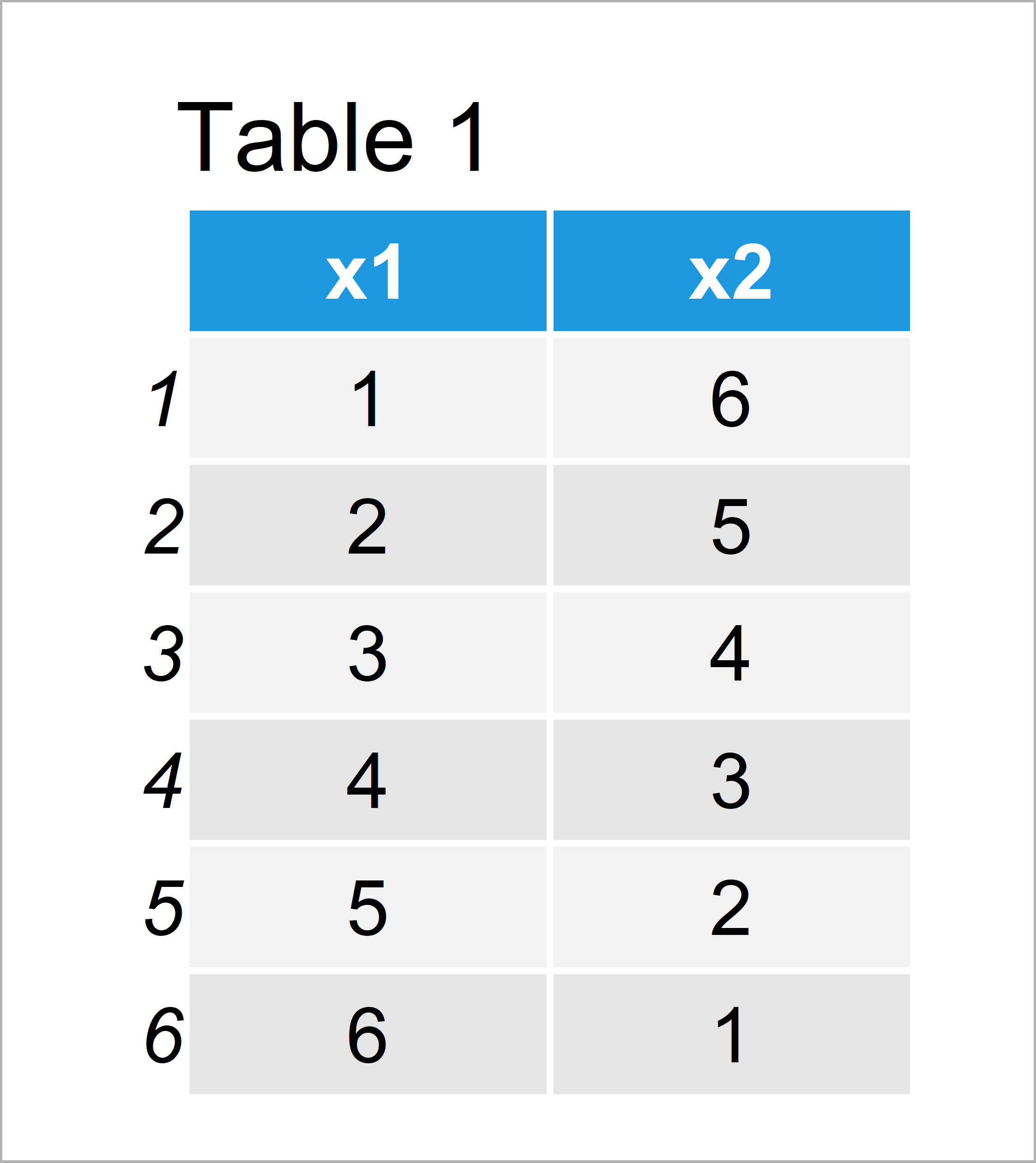Table 1 visualizes that our example data is made up of six rows and two variables.

## Example 1: Modify Color, Shape & Size of Individual Data Point in Plot Using Base R

The following code explains how to adjust the color, shape, and size of a single point in a graphic using the basic installation of the R programming language.

Let’s first draw a graph with default specifications:

`plot(data\$x1, data\$x2)          # Base R plot with default colors`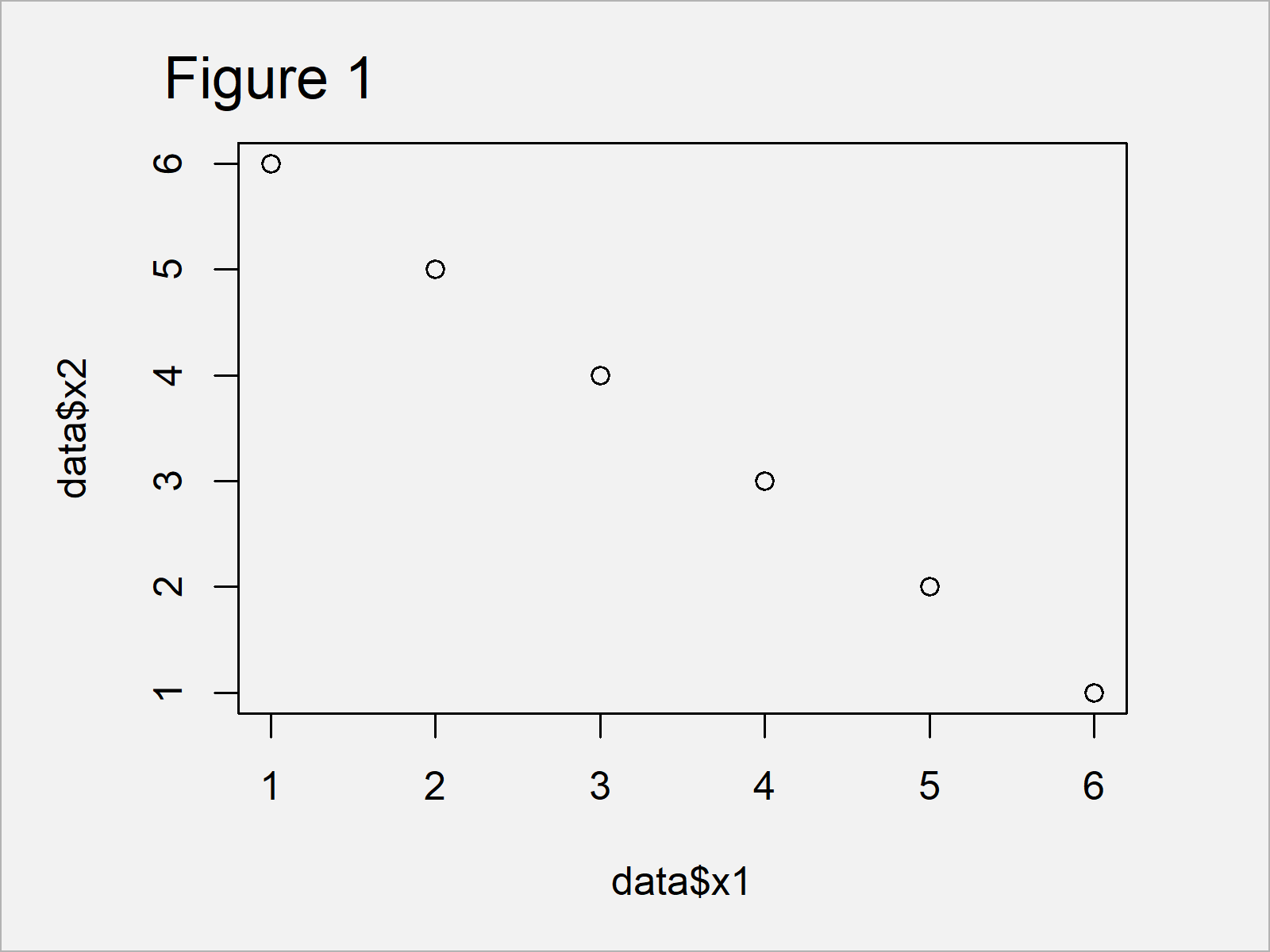Figure 1 shows the output of the previously shown syntax – A Base R scatterplot with back points only.

We can now use the ifelse and nrow functions to specify the colors (i.e. col argument), shapes (i.e. pch argument), and sizes (i.e. cex argument) of our points within the plot function.

In this example, we change these specifications for the third data point:

```plot(data\$x1, data\$x2,          # Base R plot with manual colors
col = ifelse(1:nrow(data) == 3, "red", "black"),
pch = ifelse(1:nrow(data) == 3, 15, 16),
cex = ifelse(1:nrow(data) == 3, 5, 1))```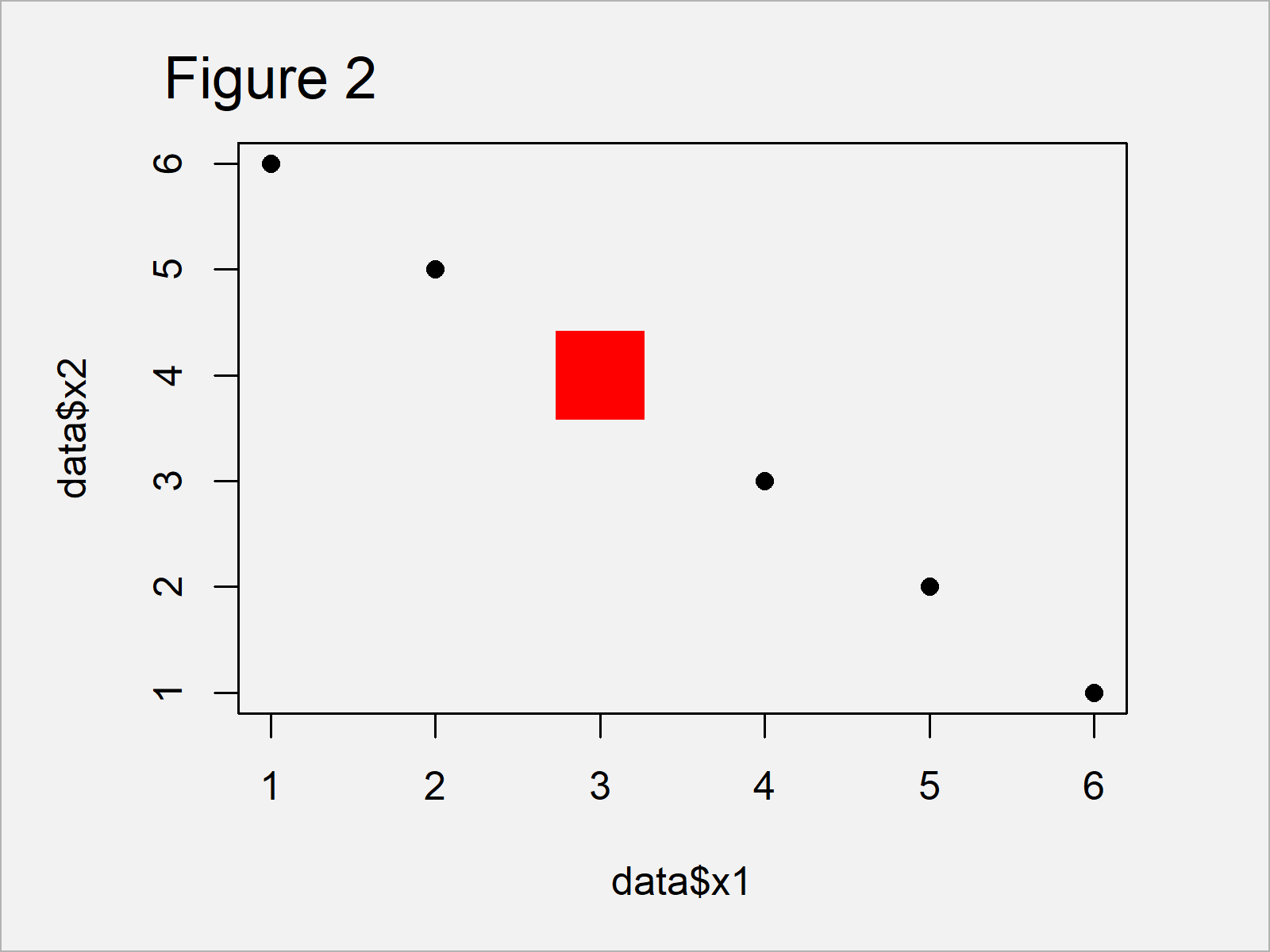As shown in Figure 2, we have created a scatterplot with different specifications for a single observation by running the previous R syntax.

## Example 2: Modify Color, Shape & Size of Individual Data Point in Plot Using ggplot2 Package

We can also change the color, shape, and size of individual points in a ggplot2 plot.

In order to use the functions of the ggplot2 package, we first need to install and load ggplot2:

```install.packages("ggplot2")     # Install & load ggplot2 package
library("ggplot2")```

Next, we can create a ggplot2 plot with default specifications:

```ggplot(data, aes(x1, x2)) +     # ggplot2 plot with default colors
geom_point()```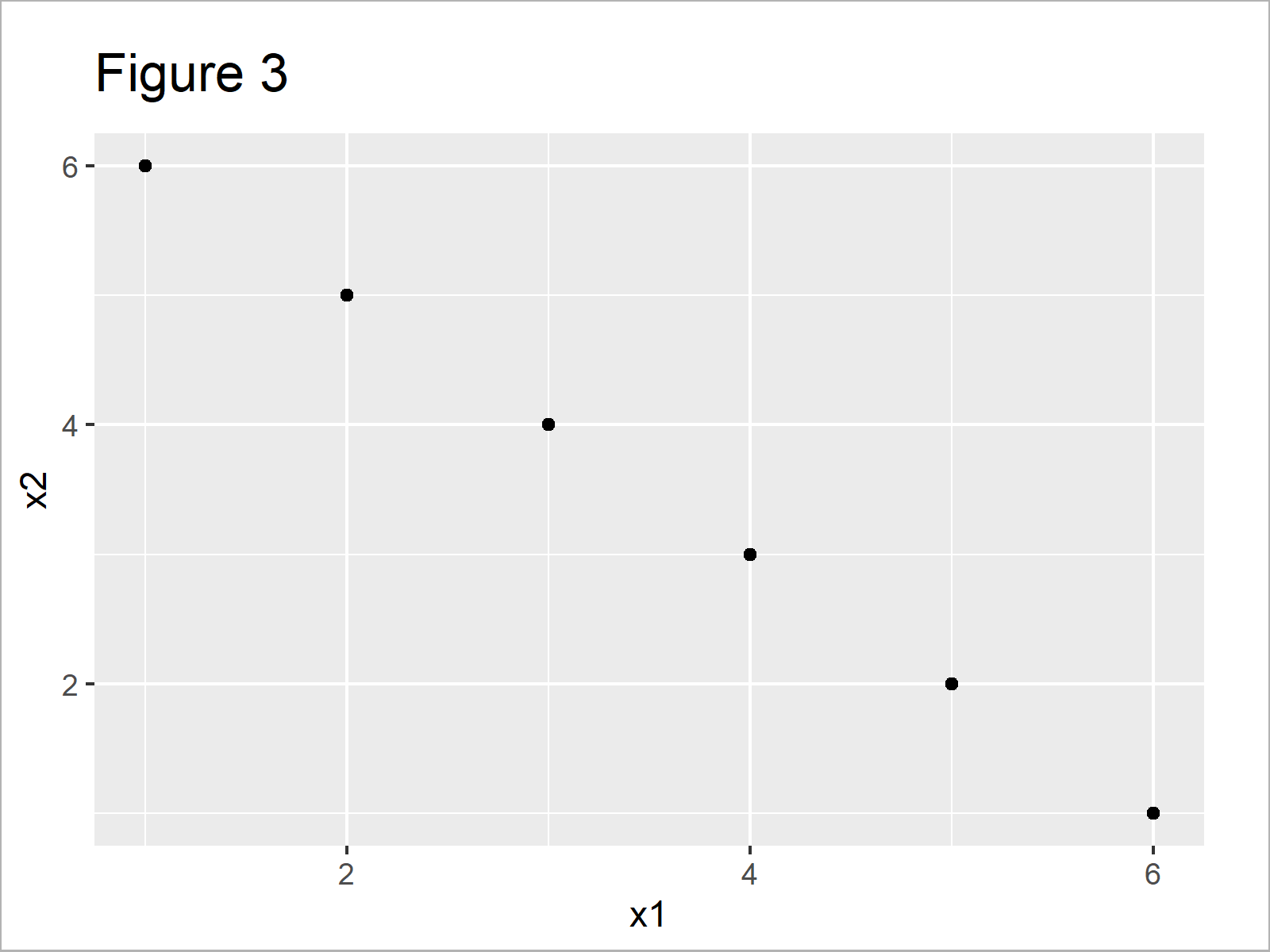As visualized in Figure 3, we have drawn a ggplot2 xy-plot without changed colors, shapes, and point sizes.

We can now adjust these attributes for one particular case by using the col, shape, and size arguments within the geom_point function:

```ggplot(data, aes(x1, x2)) +     # ggplot2 plot with manual colors
geom_point(col = ifelse(1:nrow(data) == 3, "red", "black"),
shape = ifelse(1:nrow(data) == 3, 15, 16),
size = ifelse(1:nrow(data) == 3, 5, 1))```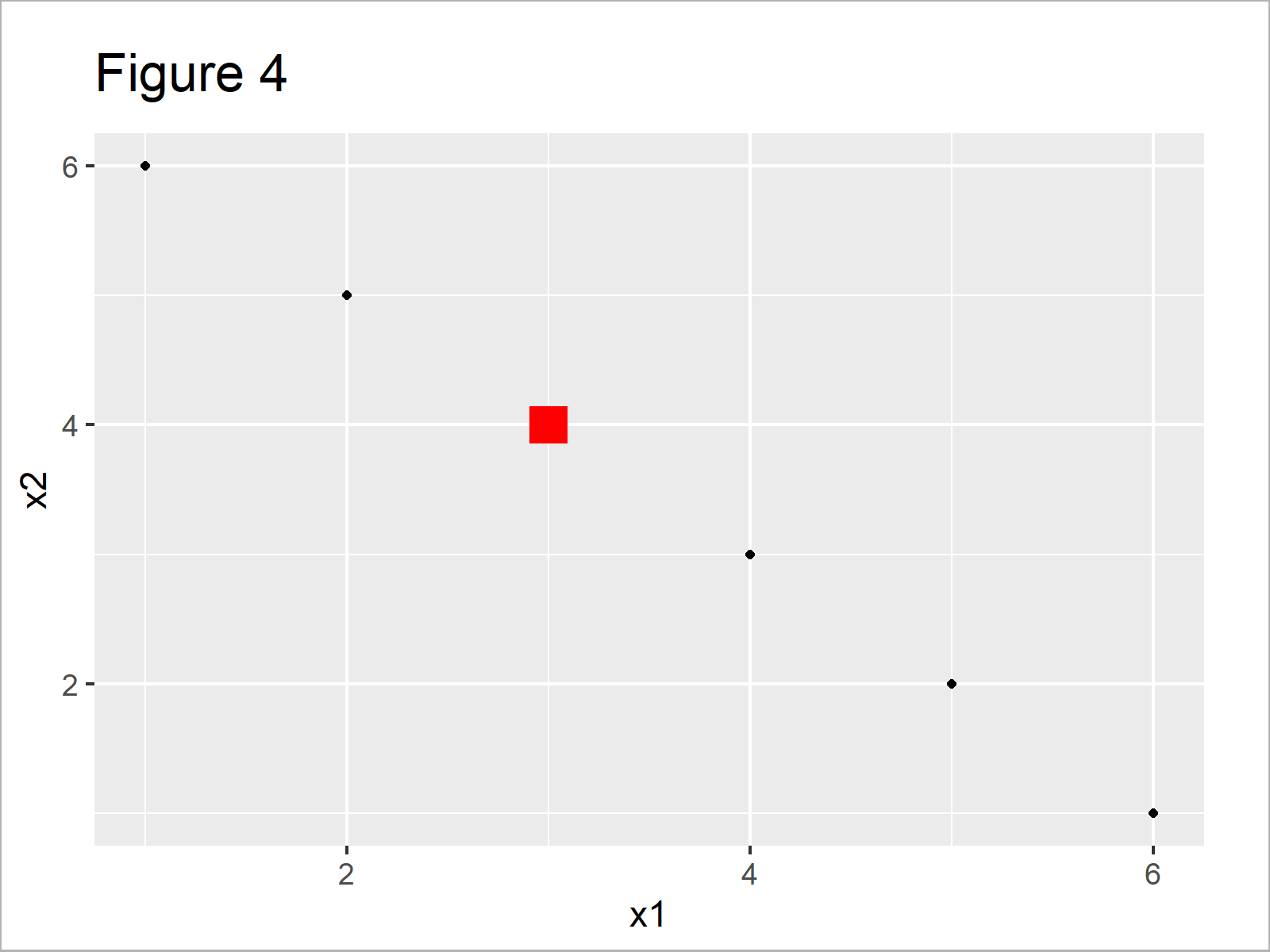After executing the previous R programming code the ggplot2 plot shown in Figure 4 has been created. As you can see, a single data point is displayed and highlighted differently compared to the other points.

## Video, Further Resources & Summary

Have a look at the following video tutorial of my YouTube channel. I’m explaining the R syntax of this page in the video:

Please accept YouTube cookies to play this video. By accepting you will be accessing content from YouTube, a service provided by an external third party.If you accept this notice, your choice will be saved and the page will refresh.

In addition, you might want to read the related tutorials of statisticsglobe.com. I have published numerous articles about topics such as ggplot2, colors, and graphics in R.

In this tutorial you have learned how to modify color, shape, and size of points in a scatterplot in the R programming language. In case you have additional questions, let me know in the comments section. Furthermore, please subscribe to my email newsletter to receive regular updates on new tutorials.

Subscribe to the Statistics Globe Newsletter